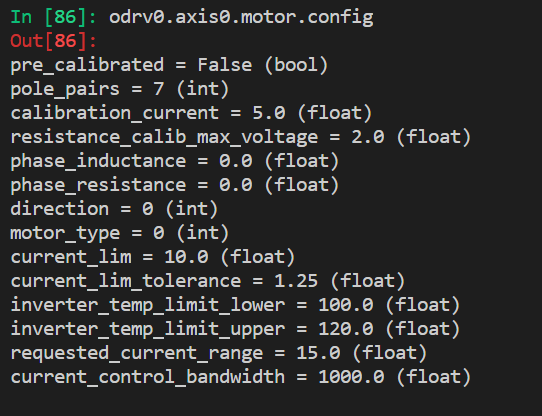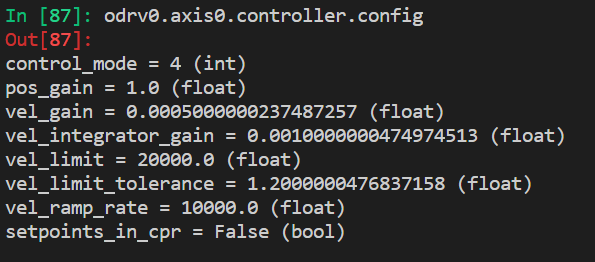# Motor only turn onece ,ang nexy not turning when after closed loop state

I have a 3.6v board and have successfully calibrated the motor and encoder. I have checked the errors before and after the closed loop state and no errors appear. But when I turn on the closed loop state the motor shakes as if trying to find the correct position and it doesn’t accept any command to rotate to a certain position. Other than that, the motor was humming.What are some possible solutions to this problem?

control_mode = 3 (int)
pos_gain = 1.0 (float)
vel_gain = 9.999999747378752e-05 (float)
vel_integrator_gain = 0.0 (float)
vel_limit = 200000.0 (float)
vel_limit_tolerance = 1.2000000476837158 (float)
vel_ramp_rate = 2000.0 (float)
setpoints_in_cpr = False (bool

Hi Kang,

First thought for me would be how solid is your encoder held in place?

If that is ok, have you run through the Setup and Tuning guide under the ODrive Documentation section? The number of poles may be out possibly.

Kind Regards,

Neil.

2 Likes

Neil ,Thanks for your reply, I am really frustrated, I still tried all kinds of ways and still can’t get my motor to work!Now the motor can go into a closed loop state, but it still can’t move.

Hi Kang,

Is there any possibility that you could share your code on here and take a short video of the motor calibrating and going in to Closed Loop Control?

Kind Regards,

Neil.

Neil！thanks for your reply! I refreshed the firmware ODrive control utility v0.4.11 ，and recalibrated it, but I found that the encoder never found the index! I used 24V 3.6 control panel, the motor is D5065 ! Here are my parameter Settings，I sincerely hope you can give me some advice！error = 0x0000 (int)
step_dir_active = False (bool)
current_state = 1 (int)
requested_state = 0 (int)
loop_counter = 8949899 (int)
lockin_state = 0 (int)
config:
startup_motor_calibration = False (bool)
startup_encoder_index_search = False (bool)
startup_encoder_offset_calibration = False (bool)
startup_closed_loop_control = False (bool)
startup_sensorless_control = False (bool)
enable_step_dir = False (bool)
counts_per_step = 2.0 (float)
watchdog_timeout = 0.0 (float)
step_gpio_pin = 1 (int)
dir_gpio_pin = 2 (int)
calibration_lockin: …
sensorless_ramp: …
general_lockin: …
motor:
error = 0x0000 (int)
armed_state = 0 (int)
is_calibrated = True (bool)
current_meas_phB = 0.28049302101135254 (float)
current_meas_phC = -0.010307461023330688 (float)
DC_calib_phB = -0.1194663867354393 (float)
DC_calib_phC = 0.21182964742183685 (float)
phase_current_rev_gain = 0.012500000186264515 (float)
thermal_current_lim = 45.52202606201172 (float)
get_inverter_temp()
current_control: …
gate_driver: …
timing_log: …
config: …
controller:
error = 0x0000 (int)
pos_setpoint = 0.0 (float)
vel_setpoint = 0.0 (float)
vel_integrator_current = 0.0 (float)
current_setpoint = 0.0 (float)
vel_ramp_target = 0.0 (float)
vel_ramp_enable = False (bool)
config: …
set_pos_setpoint(pos_setpoint: float, vel_feed_forward: float, current_feed_forward: float)
set_vel_setpoint(vel_setpoint: float, current_feed_forward: float)
set_current_setpoint(current_setpoint: float)
move_to_pos(pos_setpoint: float)
move_incremental(displacement: float, from_goal_point: bool)
start_anticogging_calibration()
encoder:
error = 0x0000 (int)
index_found = False (bool)
count_in_cpr = 6 (int)
interpolation = 0.5 (float)
phase = 0.02991262450814247 (float)
pos_estimate = 6.234378814697266 (float)
pos_cpr = 6.234375 (float)
hall_state = 3 (int)
vel_estimate = 0.0 (float)
calib_scan_response = 0.0 (float)
config: …
set_linear_count(count: int)
sensorless_estimator:
error = 0x0000 (int)
phase = 1.3786967992782593 (float)
pll_pos = 1.3817769289016724 (float)
vel_estimate = 0.1234244704246521 (float)
config: …
trap_traj:
config: …
watchdog_feed()

Hi Kang,

This is a little puzzling, not being an expert on the ODrive.

It may be worth trying to simplify it as much as possible to get confidence in each element first.

the motor must be working ok along with the 3 motor phase outputs, otherwise, I guess, it wouldn’t go into Closed Loop Control mode. I think this issue may be with the encoder, encoder cable, fixture or commands being sent.

Are you using “odrivetool” to control initially? If not, it’s worth going back to basics first such as this and then increase your systems complexity one stage at a time until you to get everything working.

have you tried sensorless control just to see if the motor rotates as you want it to?

I’ll take a snapshot of my settings too and put it on here so you have something to compare it to.

Kind Regards,

Neil.

In : odrv0.axis0.motor
Out:
error = 0x0000 (int)
armed_state = 0 (int)
is_calibrated = False (bool)
current_meas_phB = -0.014642834663391113 (float)
current_meas_phC = 0.07499802112579346 (float)
DC_calib_phB = -1.073136568069458 (float)
DC_calib_phC = -0.5179828405380249 (float)
phase_current_rev_gain = 0.02500000037252903 (float)
thermal_current_lim = 235.9759063720703 (float)
get_inverter_temp()
current_control:
p_gain = 0.015825891867280006 (float)
i_gain = 35.67265319824219 (float)
v_current_control_integral_d = 0.0 (float)
v_current_control_integral_q = 0.0 (float)
Ibus = 0.0 (float)
final_v_alpha = 0.0 (float)
final_v_beta = 0.0 (float)
Iq_setpoint = 0.0 (float)
Iq_measured = 0.0 (float)
Id_measured = 0.0 (float)
I_measured_report_filter_k = 1.0 (float)
max_allowed_current = 60.75 (float)
overcurrent_trip_level = 67.5 (float)
gate_driver:
drv_fault = 0 (int)
timing_log:
TIMING_LOG_GENERAL = 0 (int)
TIMING_LOG_MEAS_R = 0 (int)
TIMING_LOG_MEAS_L = 0 (int)
TIMING_LOG_ENC_CALIB = 0 (int)
TIMING_LOG_IDX_SEARCH = 0 (int)
TIMING_LOG_FOC_VOLTAGE = 0 (int)
TIMING_LOG_FOC_CURRENT = 0 (int)
config:
pre_calibrated = False (bool)
pole_pairs = 7 (int)
calibration_current = 20.0 (float)
resistance_calib_max_voltage = 2.0 (float)
phase_inductance = 1.5825891750864685e-05 (float)
phase_resistance = 0.03567265346646309 (float)
direction = 1 (int)
motor_type = 0 (int)
current_lim = 50.0 (float)
current_lim_tolerance = 1.25 (float)
inverter_temp_limit_lower = 100.0 (float)
inverter_temp_limit_upper = 120.0 (float)
requested_current_range = 60.0 (float)
current_control_bandwidth = 1000.0 (float)

1 Like

All of the above is working very well with the AMT 102 and the ODrive D5065 270KV.

I hope this helps.

1 Like

Thank you very much Neil ! I used the configuration you gave me, and I found that the motor works well in sensorless mode, but the encoder index is still not found. Next， I plan to replace with a new encoder, I’ll tell you ，if there is good news!

Hi Kang, no problems, happy to assist.

It may be worth connecting the encoder to a microcontroller separately, so you can see if it is the board or encoder. You could also use the ODrive for this and use the following from python command whilst turning the encoder by hand: -

start_liveplotter(lambda:[odrv0.axis0.encoder.pos_estimate,odrv0.axis1.encoder.pos_estimate])

If you swap the encoder physically from M0 to M1, the live plotter should show both inputs working and may aid diagnosis. Make sure you turn power off before swapping, it may affect something, just to be on the safe side.

It may be possible to measure the index pulse pin with a multimeter, although I haven’t looked into this.

Good luck.

2 Likes

Neil，
thanks for your valuable advice, I have solved all the problems successfully, and the motor works extremely well!I wish you good health !
Sincere blessing，
Kang.

2 Likes

Hi Kang,